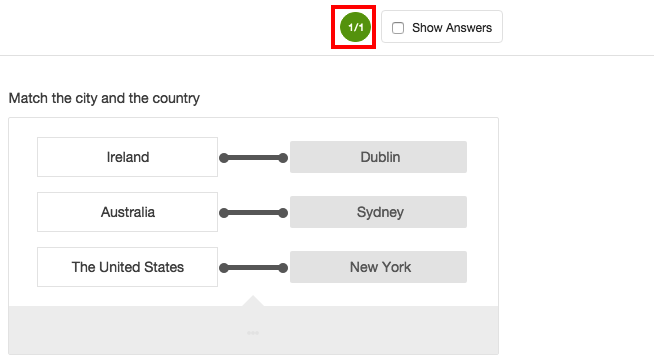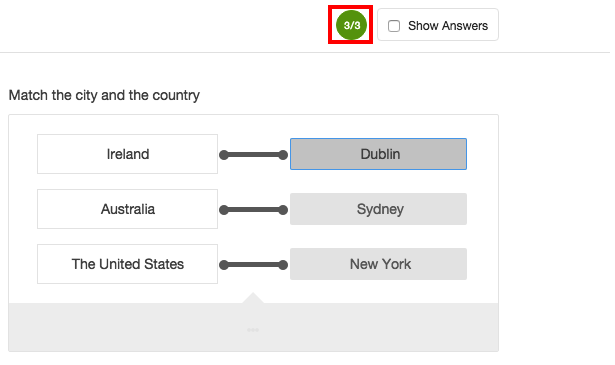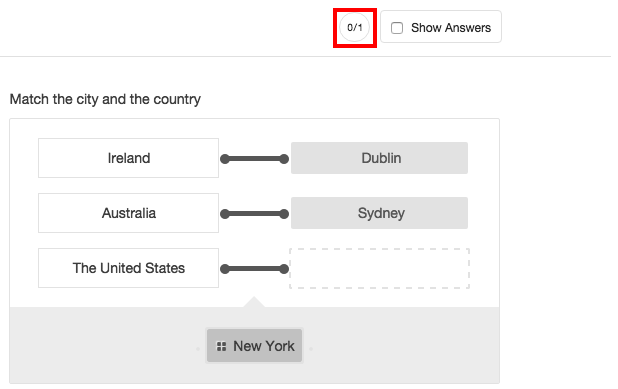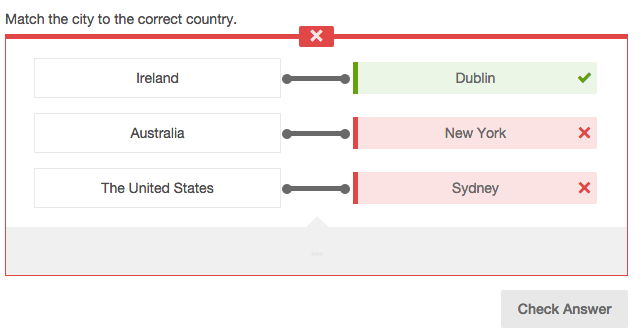# Understanding Question Validation and Scoring Types

Most of Learnosity’s Question Types are autoscored - excluding Essay, Audio and Image Highlight questions. Each auto scored Question Type has validation settings. Authors can select the scoring type, enter the points for the Question, enter a penalty score for the Question, and enable a Check Answer button.

## Scoring type

In most cases we can validate questions using 3 scoring methods: Exact Match, Partial Match Per Response, or Partial Match. Some questions, such as Classification and Order List, have additional scoring methods. More details on these can be found in tutorials for these individual Question Types.

## Exact match

The Exact Match scoring option is available in all autoscored Question Types. With Exact Match, the student must get the entire Question correct to receive a mark.Figure 1: Exact match scoring example

Figure 1 illustrates a Match List question scored using Exact Match. If each response is answered correctly, the student will receive a score of 1.

## Partial match per response

Partial scoring can be used in Questions where the student may have to answer a Question with multiple responses. Each response can be scored independently, however each response is of equal value. These scoring options are not available in all of our Question Types.

If the Question is given a score of 1, as shown in Figure 2 below, then each response within this Question will be worth 1 mark. As a result, a possible max score for the Question will be 3.Figure 2: Partial match per response scoring example

## Partial match

Partial Match is similar to Partial Match per Response. A student gets a partial score for each correct response within the Question. The difference with Partial Match is that the value entered in the Points box is the overall mark for the Question.

If the Question is given a score of 1, as shown in Figure 3 below, then each response within this Question will be worth .3333 (rounded to 4 decimal places).Figure 3: Partial match scoring example

Under Partial Match there is also a Rounding option. Rounding is only available when the scoring type Partial Match has been selected. There are two options to select from the drop down menu: None or Floor. None is set as the default option.

• None will round the score to four decimal places e.g. 2.6666
• Round down will round the score down to the nearest whole number, e.g. a score of 2.666 will be rounded down to 2.

## Penalty score

This will determine if marks are deducted for an incorrect answer. By default, the Penalty Score is set to 0. In the Match List examples above, there are 3 responses to the Question. If we apply a penalty score of 1 to the examples shown above, then:

• Exact Match - If the student answers any of the responses incorrectly, they will receive a score of -1 for the Question.
• Partial Match Per Response - For each incorrect response, the student will receive a score of -1, with a possible max penalty score of -3.
• Partial Match - For each incorrect response, the student will recieve a score of -.3333 with a max penalty score of -1. If rounding has been enabled, it will also apply to the penalty score.

Check Answer is a button that appears below a Question, and allows students to check if their response is correct. It is usually only applied to formative assessments. Check Answer can be enabled by toggling the Show Check Answer Button option in the Question Editor. Feedback attempts can be configured to allow the student to test as many times as needed. The default setting is unlimited attempts. The Check Answer button will not disclose the correct answer, but will indicate which answers have been answered incorrectly as shown in Figure 4.## Non autoscored questions

Essay (including Math and Chemistry), Audio and Image Highlight Question Types need to be scored by a teacher. While a correct answer cannot be specified in the Question Editor, a maximum score can be set. The option can be found under More Options accordion and is developer only.

## Unscored questions

The Unscored/Practice Usage option allows authors to disable scoring for a question. When enabled, the question will receive no score. This can be found in the scoring section of a question